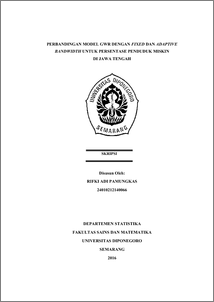# PERBANDINGAN MODEL GWR DENGAN FIXED DAN ADAPTIVE BANDWIDTH UNTUK PERSENTASE PENDUDUK MISKIN DI JAWA TENGAH

PAMUNGKAS, RIFKI ADI (2016) PERBANDINGAN MODEL GWR DENGAN FIXED DAN ADAPTIVE BANDWIDTH UNTUK PERSENTASE PENDUDUK MISKIN DI JAWA TENGAH. Undergraduate thesis, Fakultas Sains dan Matematika.Preview
PDF
3591Kb

## Abstract

Regression analysis is statistical method for modeling the dependency relationship that might exist among the dependent variable with independent variable. Geographically Weighted Regression (GWR) is an expansion of linier regression model where each of the parameters from every observation sites is counted, so each sites have local regression parameter. Weighted Least Square (WLS) model is applied to estimate the parameter of GWR model. GWR method differentiates bandwidth kernel into two, fixed bandwidth kernel and adaptive bandwidth kernel. Fixed kernel has the same bandwidth in each observation location, meanwhile adaptive kernel has different bandwidth value in each observation location. Cross Validation (CV) is used to choose the most optimum bandwidth. The application of GWR model to show the percentage of poor population at district and city of Central Java shows that GWR model is significantly different in each location towards global regression model, also the estimated model will also give different result between one location and another. Based on Akaike Information Criterion (AIC) value between global regression models with GWR, it is know that GWR model with fixed exponentially weighted kernel is the best model to use to analyze the percentage poor population at district and city of Central Java because of it has the smallest AIC value. Keywords: Akaike Information Criterion, Bandwidth, Cross Validation, Exponential Kernel Function, Geographically Weighted Regression, Weighted Least Square

Item Type: Thesis (Undergraduate) H Social Sciences > HA Statistics Faculty of Science and Mathematics > Department of Statistics 55018 Mr Hasbi Yasin 25 Jul 2017 09:12 25 Jul 2017 09:12

Repository Staff Only: item control page